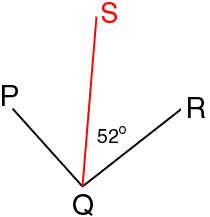Name: monica Who is asking: Student Level: Secondary Question: Ray QS is the bisector for angle PQR. Find the measure of angle PQS and PQR if the measure of angle SQR is 52 degrees. Hi Monica, Since QS is a bisector of angle PQR, the measures of the angles PQS and SQR are equal. But the measure of angle SQR is 52 degrees and hence the measure of angle PQS is also 52 degreesFrom the diagram the measure of the angle PQR is the measure of PQS plus the measre of SQR. Cheers, Penny Go to Math Central